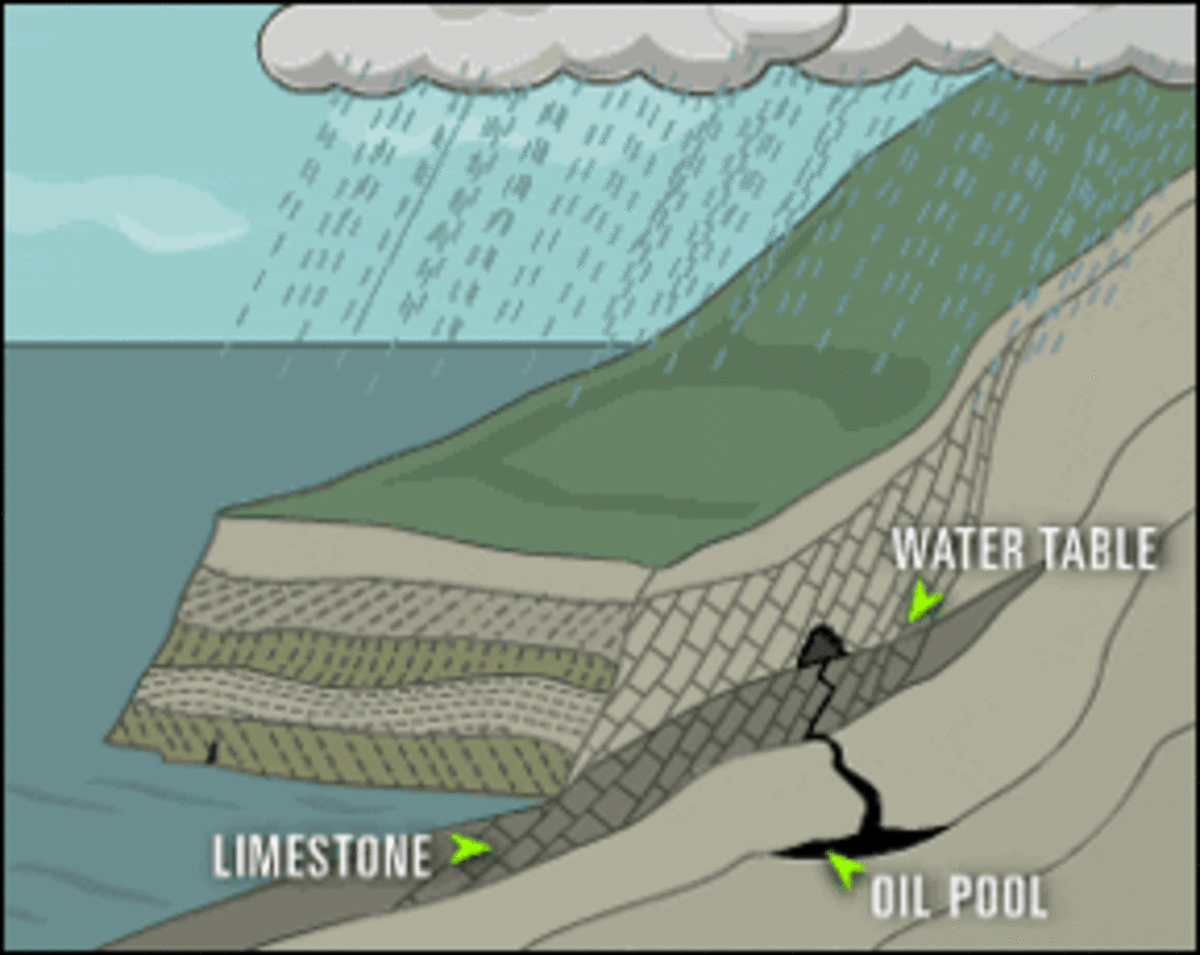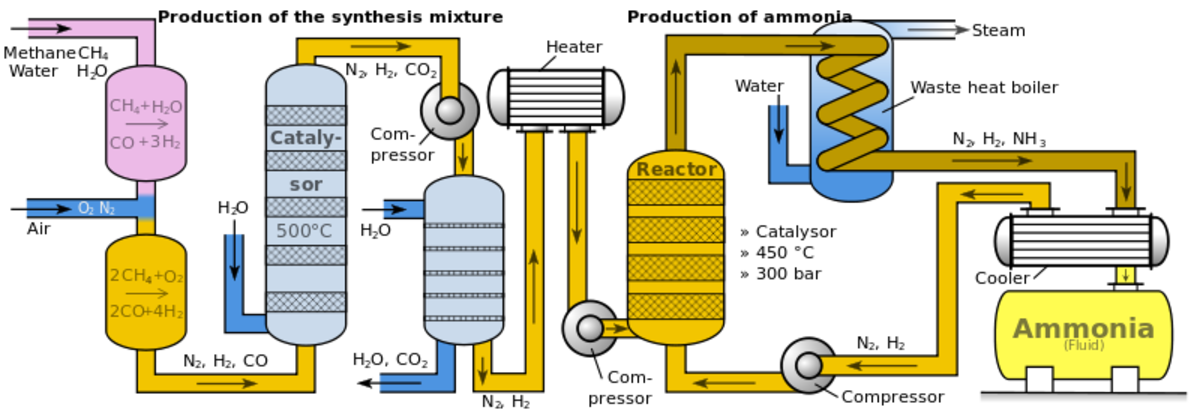# Chemical Equilibrium Equations and Equilibrium Constants Formula (Haber Bosch Process, limestone cave formation)

[EDITOR'S NOTE: Other exam topics are: Macromolecules and Polymers, Acid/Base reactions, Redox reactions, environmental chemistry (ozone depletion), Molecules/Salts/Metals/Atomic lattice, Equilibrium equations and organic chemistry]

## Equilibrium constant and reaction formula

Equilibrium equations in chemistry define which side of a reaction dominates (reactant side or product side). Depending which side is stronger the reaction is more or less likely to happen.
In the first part, you will learn about the basics and the second part will consist of examples, where equilibrium equations appear in everyday life and nature.

The general form of an equilibrium equation is:

aA + bB ↔ cC + dD
The capital letters A, B, C and D stand of the reactants and products, whereas the small letters a, b, c and d are the so-called stoichiometric coefficients in the balanced equation.

In order to find out the concentration of the reactants and products, the following general equation is used.

Keq = [product]x / [reactant]y

Keq signifies a constant number in the equilibrium equation and remains therefore unchanged.
The words (or molecules) in the square parentheses stand for the concentration of the molecules given in moles per gram. The small letters x and y are variables which can be a, b, c or d.
To make this clearer, the formula above will be applied on an example.
Keep in mind that these equations are only applied on gaseous or aqueous (solved) molecules. Solids and liquids are assumed to have a constant concentration and do not appear in these equations. Should they appear in any case, they can be “ignored”.

1N2 (g) + 3H2 2NH3 (g) (+heat)

The equation Keq = [product]x / [reactant]yis applied in order to find out the concentrations.
The product is 2NH3 and the reactants are N2 and 3H2, so:

Keq = [NH3]2 / [N2]1 * [H2]3

As one can see the molecules used in the first equation are the powers of the concentration values in the second equation. Imagine you used 3 hydrogen molecules and one nitrogen molecule. So, there was three times more hydrogen concentrated in the first part than nitrogen. So, you calculate
[H2] * [H2] *[H2] = [H2]3. Therefore, the ration used and achieved (on the product side) tells you also the power of the concentration.Acidic rain falls on the ground. The water penetrates the soil and reacts with the limestone in the

## Equilibrium equations in nature: formation of limestone caves

As one can see it on the picture; the ground consists of CaCO3 or limestone in other words.
Acidic rain falls down on the earth and an acid / base reaction takes place:
Formation of acidic rain: CO2 + H2O ↔ H2CO3
H2CO3 is a very acidic compound and will behave as an acid during the limestone formation.
The acidic rain (H2CO3) dissolves the limestone:
H2CO3 (g) + CaCO3 (s) ↔ 2HCO3- (aq) + Ca2+ (s)
CO32- behaved as a base. For the calculation of the concentrations Calcium can be neglected since it is a solid.
The equilibrium is constantly shifting (in nature) and is also responsible for the formation of stalagmites and stalactites.

Scroll to Continue

## Equilibrium equations in daily life: Formation of Fizzy Powder (e.g. froth of Coca-Cola)

The formation of froth or fizzy powder is the spontaneous decay of carbonic acid into water and carbon dioxide.

H2CO3 (aq) ↔ H2O (aq) + CO2 (aq)
They are all aqueous (aq), because they are in a solution.

## Equilibrium equations in Industry: Haber-Bosch-Process

Haber-Bosch-procedure: The Haber-Bosch-procedure is the process of gathering ammonia where it is used in various fields. The general equation is:
N2+3H2 ↔ 2NH3
But in order to obtain NH3,or ammonia, the reaction has several immediate steps in order to catalyse this equation. Without any catalysts, nitrogen will not react with hydrogen because large amount of energy is needed to break the triple bond.
. That is why this reaction is highly exothermic.
The N2 or nitrogen goes into a catalyst, which is a reactor and a compressor. In this first step, nitrogen experiences a high temperature (about 300°C to 550°C) and a very high pressure (150-300bar). Afterwards it passes the heat recovery boiler as a hydrogen-nitrogen-ammonia mixture, where it will cool down and then goes along to the gas recovery part, where the final product ammonia (NH3) is collected. The cooling part is necessary in order to maintain a reasonable equilibrium constant.Wikipedia, only right side of the heater is relevant for the text above.